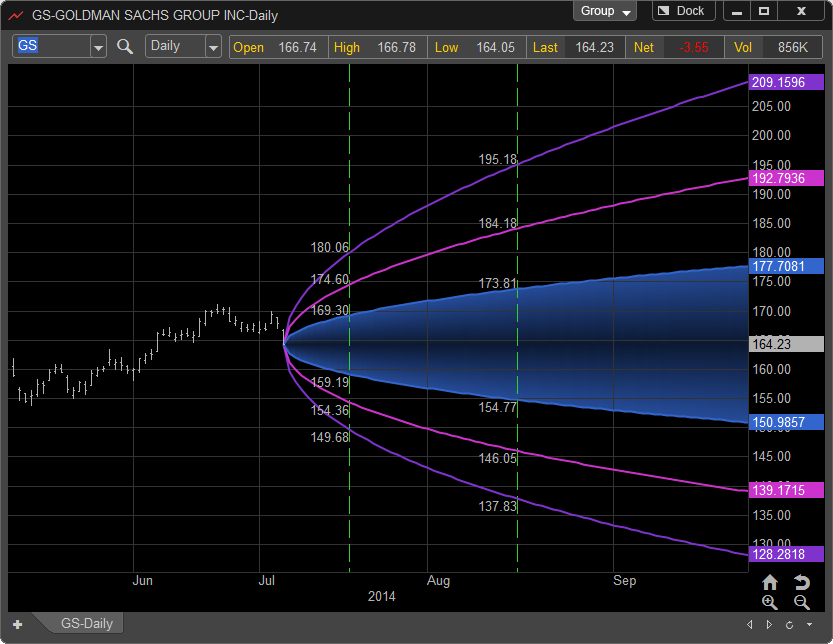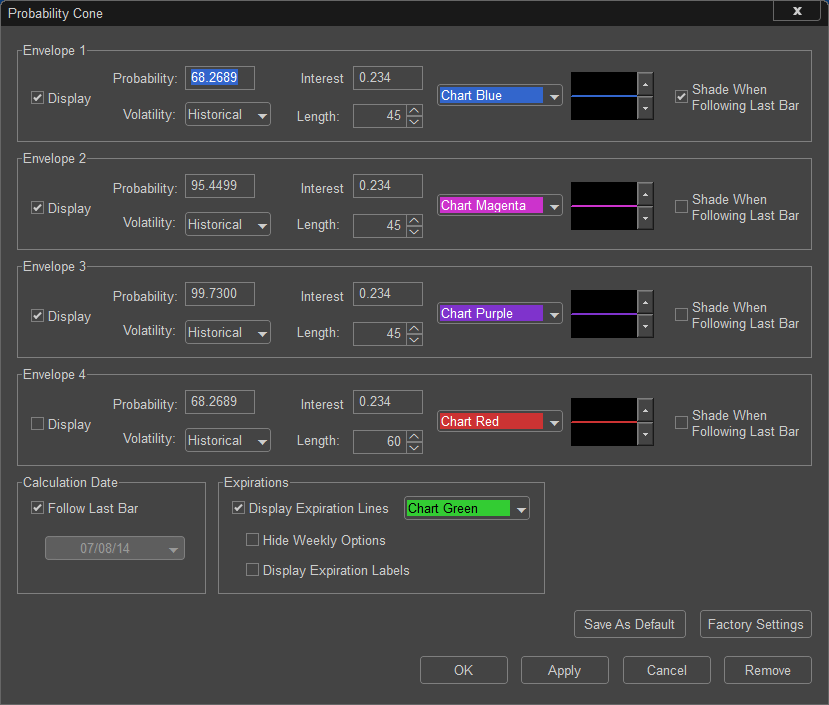# Probability Cone

The main purpose of the Probability Cone is to provide a graphic representation of odds for an individual market to reach a price and time projection.  For example, we have a Probability Cone set to GS using the Options Analytix default settings.  The default settings use a 45 period Historical Volatility calculated from the current price with the Probability's set to One, Two, and Three Standard Deviations (68.2689%, 95.4499%, and 99.73%).

To interpret the Probability Cone in its’ most simple form you first identify where you assume the price of the market will be on a future date and the Cone will give you the estimated percentage of probability that prices will be there.  For example, any price in the future that occurs between the Blue Shaded area of the Cone (white shaded area) has a 68.2689% chance of occurring based on historical volatility.  As the time range moves into the future the cones or area of potential price movement grow larger.  To aid in the use of the probabilities around option expiration the Probability Cone displays future option expirations as green dashed vertical lines.

The theory behind this is that a market has a much greater chance of trading at a price range \$40 dollars above or below the current price in 6 months than it does of only a \$5 dollar range based on the options pricing methods.Probability Cone Setting Dialog: